# NR SRS Configuration

This example shows how to generate sounding reference signals (SRS), as defined in TS 38.211 Section 6.4.1.4 , including SRS configuration, symbol and indices generation, OFDM resource grid mapping, and SRS waveform generation. The example demonstrates how to select the appropriate parameters to position the SRS in frequency and to setup:

• Full-band transmissions: Generate an SRS spanning all the available bandwidth.

• Frequency-hopping transmissions: Generate periodic and aperiodic SRS transmissions with different frequency-hopping patterns.

• Multi-user transmissions: Generate orthogonal SRS using time, frequency, and cyclic shifts.

### Introduction

Sounding reference signals are uplink physical signals employed by user equipment (UE) for uplink channel sounding, including channel quality estimation and synchronization. Unlike demodulation reference signals (DM-RS), SRS are not associated to any physical uplink channels and they support uplink channel-dependent scheduling and link adaptation. SRS assist in:

• Codebook-based closed-loop spatial multiplexing.

• Reciprocity-based downlink precoding in multi-user MIMO setups.

• Quasi co-location of physical channels and reference signals.

### Frequency Positioning

This section shows how to configure the SRS position in frequency domain and determine the bandwidth allocated to the SRS.

Configure a 15 MHz bandwidth carrier with 15 kHz subcarrier spacing (SCS).

```carrier = nrCarrierConfig; carrier.NSizeGrid =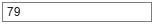79; % Bandwidth in RB carrier.SubcarrierSpacing =15;```

The bandwidth configuration parameters `CSRS` and `BSRS` control the bandwidth allocated to the SRS, which normally increases with `CSRS` and decreases with `BSRS`. Use these interactive controls to configure the SRS bandwidth.

```srs = nrSRSConfig; srs.CSRS =10; % Bandwidth configuration C_SRS (0...63) srs.BSRS =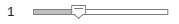1; % Bandwidth configuration B_SRS (0...3)```

To modify the frequency position of the SRS, change the values of `FrequencyStart` and `NRRC`. `FrequencyStart` specifies the frequency origin of the SRS in RBs with respect to the carrier origin when `NRRC = 0`.

```srs.FrequencyStart =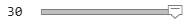30; % Frequency position of the SRS in carrier in RB (0...271) srs.NRRC =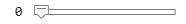0; % Frequency domain position in blocks of 4 PRB (0...67) hSRSGrid(carrier,srs,1,true); % Create and display a single-slot resource grid containing SRS title(['Resource Grid Containing SRS. NRRC = ' num2str(srs.NRRC)]); hSRSAnnotations(carrier,srs);```This figure displays a single-slot OFDM resource grid containing an SRS. For `CSRS = 10` and `BSRS = 1`, the frequency position of the SRS $\left({\mathit{F}}_{0}\right)$ shifts by ${\mathrm{NRB}}_{\mathit{T}}$ when `NRRC` is in the range (3...5) and by $2{\mathrm{NRB}}_{\mathit{T}}$ when `NRRC` is in the range (6...8). The SRS returns to the initial position (`FrequencyStart`) when `NRRC` is 9. ${\mathrm{NRB}}_{\mathit{T}}$ is the number of resource blocks (RBs) allocated to the SRS per transmission.

This figure illustrates the concepts introduced above for `CSRS = 10` and `BSRS = 1`.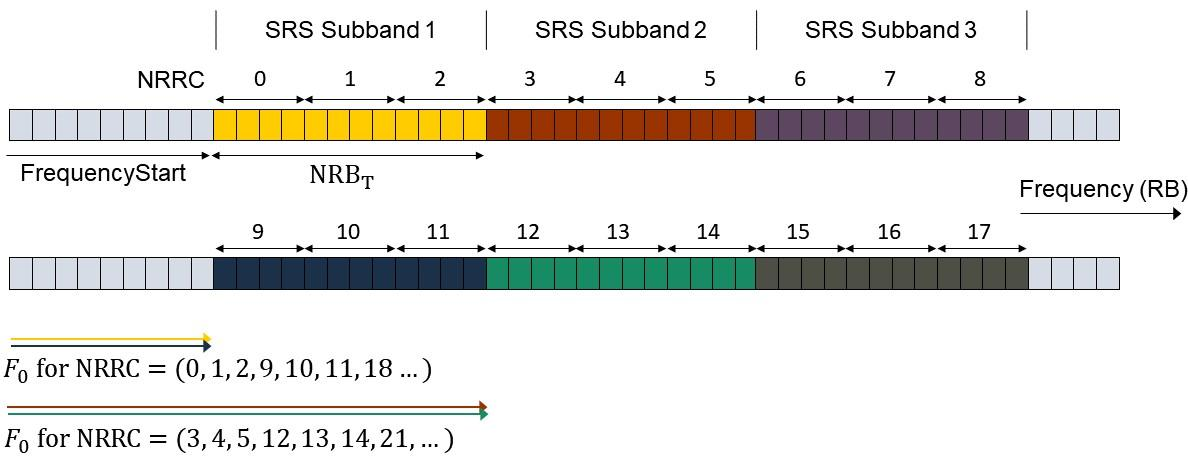`NRRC` is an additional frequency offset specified as a number of 4 RBs and it corresponds to the higher layer parameter freqDomainPosition (see TS 38.331 Section 6.3.2 SRS-Config ). For values of `NRRC` between $\mathit{k}\cdot \left({\mathrm{NRB}}_{\mathit{T}}/4\right)$ and $\left(\mathit{k}+1\right)\cdot \left({\mathrm{NRB}}_{\mathit{T}}/4\right)-1$, the frequency position of the SRS is shifted by ${\mathit{k}\cdot \mathrm{NRB}}_{\mathit{T}}$, where $\mathit{k}$ is an integer. TS 38.211 Section 6.4.1.4 refers to ${\mathrm{NRB}}_{\mathit{T}}$ as ${\mathit{m}}_{\mathrm{SRS}.\mathit{b}}$ with $b=\mathrm{BSRS}$. For more information, see the `NRBPerTransmission` property of the `nrSRSConfig` configuration object.

This equation determines the origin of the SRS in frequency:

`${\mathit{F}}_{0}=\mathrm{FrequencyStart}+{\mathrm{NRB}}_{\mathit{T}}\cdot \mathrm{mod}\left(⌊4\frac{\mathrm{NRRC}}{{\mathrm{NRB}}_{\mathit{T}}}⌋,\mathrm{NSB}\right)$`

$\mathrm{NSB}$ denotes the number of SRS subbands (frequency bands of size ${\mathrm{NRB}}_{\mathit{T}}$) where the SRS can be positioned through the parameter `NRRC`. To calculate $\mathrm{NSB}$, you can use the SRS bandwidth configuration table (see TS 38.211 Table 6.4.1.4.3-1). You can also access this table through the `BandwidthConfigurationTable` property of the `nrSRSConfig` object.

```csrs = srs.CSRS; disp(nrSRSConfig.BandwidthConfigurationTable(csrs+(0:2) + (csrs==0),:));```
``` C_SRS m_SRS_0 N_0 m_SRS_1 N_1 m_SRS_2 N_2 m_SRS_3 N_3 _____ _______ ___ _______ ___ _______ ___ _______ ___ 9 32 1 16 2 8 2 4 2 10 36 1 12 3 4 3 4 1 11 40 1 20 2 4 5 4 1 ```

The first column contains possible values of the parameter `CSRS`. For `CSRS = 10` and `BSRS = 1`, the number of unique SRS subbands is $\mathrm{NSB}={\mathit{N}}_{0}\cdots {\mathit{N}}_{{\mathit{B}}_{\mathrm{SRS}}}=3$, where ${\mathit{N}}_{0}=1$ and ${\mathit{N}}_{1}=3$.

```% Calculate and display the number of SRS subbands configurable by NRRC NSBTable = hSRSNumberOfSubbandsOrHoppingPatterns(srs); fprintf('Number of SRS subbands (NRRC < %d): %d', NSBTable*srs.NRBPerTransmission/4,NSBTable);```
```Number of SRS subbands (NRRC < 9): 3 ```
```% Calculate the frequency origin of the first SRS symbol f0 = hSRSFrequencyOrigin(srs); fprintf('The frequency origin of the SRS is F0 = %d RB.', f0);```
```The frequency origin of the SRS is F0 = 30 RB. ```

Confirm the frequency position of the SRS using the `info` output of nrSRSIndices

```[~,info] = nrSRSIndices(carrier,srs); display(info.PRBSet(1))```
``` 30 ```

For a given value of `CSRS`, the frequency band where the SRS can be allocated using `NRRC` is $\mathrm{FrequencyStart}+\left(0\dots \mathrm{NSB}\cdot {\mathrm{NRB}}_{\mathit{T}}-1\right)$. For `CSRS = 10`, the SRS wraps around when $\mathrm{NRRC}=\mathrm{NSB}\cdot {\mathrm{NRB}}_{\mathit{T}}/4={\mathit{N}}_{0}\cdots {\mathit{N}}_{{\mathit{B}}_{\mathrm{SRS}}}\cdot {\mathit{m}}_{\mathrm{SRS},\mathit{b}}/4={\mathit{m}}_{\mathrm{SRS},0}/4=9$ , which results into the same frequency position as that of $\mathrm{NRRC}=0$.

`fprintf('The SRS frequency range is limited to the range (%d,%d) RB for the current value of CSRS (%d).',srs.FrequencyStart,srs.FrequencyStart + NSBTable*srs.NRBPerTransmission,srs.CSRS);`
```The SRS frequency range is limited to the range (30,66) RB for the current value of CSRS (10). ```

When intra-slot frequency hopping is enabled, the calculation of `FrequencyOrigin` is only valid for the first SRS symbol in the slot. For frequency-hopping SRS, use the outputs `info.PRBset `or `info.SubcarrierOffset` of the `nrSRSIndices` function to determine the frequency position of subsequent symbols.

`fprintf('The frequency origin of the SRS is F0 = %d RB.',info.PRBSet(1));`
```The frequency origin of the SRS is F0 = 30 RB. ```

### Full-Bandwidth Configuration

This section shows how to configure and generate a full-band SRS transmission by calculating the appropriate SRS bandwidth parameters for a carrier.

Configure a 10 MHz bandwidth carrier with 15 kHz SCS.

```carrier = nrCarrierConfig; carrier.NSizeGrid =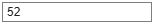52; carrier.SubcarrierSpacing =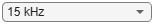15;```

The bandwidth configuration parameters `CSRS`, `BSRS`, and `BHop` control the bandwidth allocated to the SRS. To configure a full-band SRS, set `CSRS = 14` and `BSRS = 0`.

```srs = nrSRSConfig; srs.CSRS =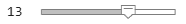13; % Bandwidth configuration C_SRS (0...63) srs.BSRS =1; % Bandwidth configuration B_SRS (0...3) hSRSGrid(carrier,srs, 1, true); % Create and display a single-slot resource grid containing SRS title(['Resource Grid with Full-Band SRS. CSRS = ' num2str(srs.CSRS)]); hSRSAnnotations(carrier,srs);```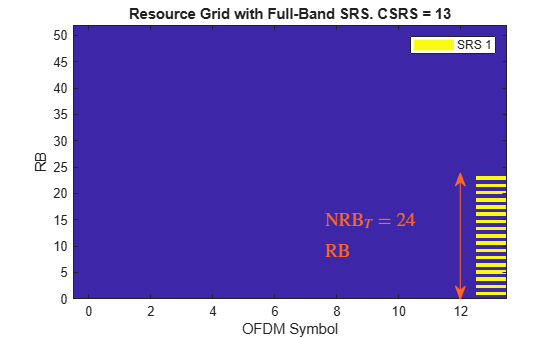For an SRS frequency allocation, you can find the appropriate values of `CSRS`, `BSRS`, and `BHop` in the SRS bandwidth configuration table (see TS 38.211 Table 6.4.1.4.3-1). Alternatively, `nrSRSConfig.BandwidthConfigurationTable` provides an easy way to access and display this table.

```% To display relevant rows of the bandwidth configuration table, calculate the value of CSRS for a full band SRS [csrs,bsrs] = hSRSBandwidthConfiguration(srs,carrier.NSizeGrid); % Display bandwidth configuration table disp(nrSRSConfig.BandwidthConfigurationTable(csrs+(0:2) + 1*(csrs==0),:));```
``` C_SRS m_SRS_0 N_0 m_SRS_1 N_1 m_SRS_2 N_2 m_SRS_3 N_3 _____ _______ ___ _______ ___ _______ ___ _______ ___ 13 48 1 24 2 12 2 4 3 14 52 1 4 13 4 1 4 1 15 56 1 28 2 4 7 4 1 ```

The columns labeled as `m_SRS_b` with `b `= 0...3 contain the number of RBs allocated to the SRS for the parameter `b = BSRS` and non-hopping configurations. The row corresponding to `C_SRS = 14` contains a number of RBs `m_SRS_0 = 52`, which is the closest value to the carrier bandwidth. Therefore, the parameters `CSRS = 14` and `BSRS = 0` configure a full-band SRS transmission for the current carrier configuration. This example uses the bandwidth configuration table to calculate the values of `CSRS` and `BSRS` that maximize the SRS bandwidth within the carrier.

`fprintf('For a full-band SRS in a carrier bandwidth of %d RB, set CSRS = %d and BSRS = %d.',carrier.NSizeGrid,csrs,bsrs);`
```For a full-band SRS in a carrier bandwidth of 52 RB, set CSRS = 14 and BSRS = 0. ```

You can use the SRS read-only property `NRBPerTransmission` to confirm that the generated SRS fits into the carrier bandwidth.

`fprintf('The SRS bandwidth (%d RB) is lower than or equal to the carrier bandwidth (%d RB).',srs.NRBPerTransmission,carrier.NSizeGrid);`
```The SRS bandwidth (24 RB) is lower than or equal to the carrier bandwidth (52 RB). ```

### Frequency-Hopping Configuration

You can configure intra-slot and inter-slot frequency hopping for multi-symbol and multi-slot SRS transmissions, respectively. The instantaneous bandwidth per OFDM symbol is constant across SRS OFDM symbols and is smaller than the bandwidth over which the SRS hops.

Configure a 15 MHz bandwidth carrier with 15 kHz SCS.

```carrier = nrCarrierConfig; carrier.NSizeGrid =79; carrier.SubcarrierSpacing =15;```

Create a four-symbol SRS located at the end of the slot. Select the repetition factor to indicate the number of equal consecutive SRS transmissions (OFDM symbols) in a slot. For frequency-hopping configurations, `Repetition` must be lower than the number of SRS symbols.

```srs = nrSRSConfig; srs.NumSRSSymbols =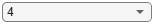4; srs.Repetition =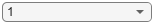1; srs.SymbolStart =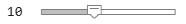10; % Time-domain position of the SRS in the slot. (8...13) for normal cyclic prefix (CP) and (6...11) for extended CP```

Downlink control information (DCI) can trigger aperiodic SRS transmissions using the higher layer parameter resourceType (see TS 38.331 Section 6.3.2 SRS-Config). As the frequency hopping pattern is reset for aperiodic SRS resource types at each slot, select `periodic` or `semi-persistent` SRS resource types to enable inter-slot frequency hopping or `aperiodic` to disable it. You can configure the slot-wise periodicity and offset of the SRS transmissions by using the property `SRSPeriod`. For `aperiodic` resource types, the parameter `SRSPeriod` controls the periodicity and offset of DCI signals triggering aperiodic SRS transmissions.

```srs.ResourceType =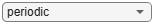"periodic"; srs.SRSPeriod = [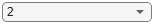2 0]; % Periodicity in slots (1,2,4,5,8,10,...) srs.SRSPeriod(2) =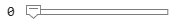0; % Offset in slots must be smaller than the periodicity```

Use these interactive controls to select a hopping configuration and observe the changes to the OFDM resource grid.

```srs.CSRS =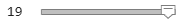19; % Bandwidth configuration C_SRS (0...63) srs.BSRS =2; % Bandwidth configuration B_SRS (0...3) srs.BHop =0; % Frequency hopping configuration (0...3). Set BHop >= BSRS to disable frequency hopping srs.NRRC =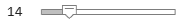14; % Frequency domain position in blocks of 4 PRB (0...67) % Create and display a multi-slot resource grid containing SRS duration = 2*srs.SRSPeriod(1); % Transmission length in slots hSRSGrid(carrier,srs, duration, true); title('Carrier Grid Containing Frequency-Hopping SRS')```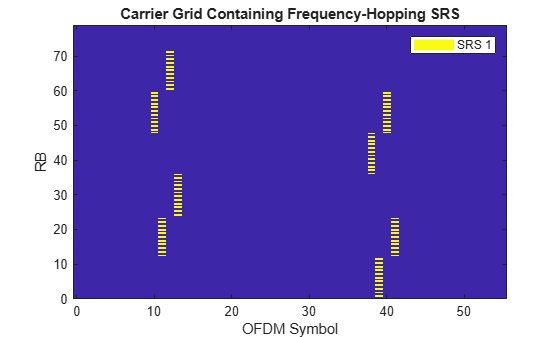The bandwidth over which the SRS symbols hop increases with the value of `CSRS` and decreases with `BHop` (until `BHop = BSRS` disables hopping). Increasing `BSRS` reduces the allocated bandwidth per OFDM symbol (property `NRBPerTransmission`) and can reduce the intra-slot frequency hopping as well. To disable frequency hopping, set `BHop >= BSRS`. For non-hopping configurations, the roles of `CSRS` and `BSRS` are analogous, as the allocated bandwidth increases with `CSRS` and decreases with `BSRS`.

You can use the bandwidth configuration table (TS 38.211 Table 6.4.1.4.3-1) to calculate the number of different frequency-hopping patterns configurable by the SRS parameter `NRRC` as $\mathrm{NFHP}={\mathit{N}}_{\mathrm{BHop}+1}\cdots {\mathit{N}}_{{\mathit{B}}_{\mathrm{SRS}}}$. The frequency-hopping patterns repeat when $\mathrm{NRRC}>\mathrm{NFHP}\cdot {\mathrm{NRB}}_{\mathit{T}}/4$.

```N = hSRSNumberOfSubbandsOrHoppingPatterns(srs); if srs.BHop < srs.BSRS && srs(1).NRB > 16 % Number of unique frequency-hopping patterns fprintf('Number of unique frequency-hopping patterns (configurable by NRRC < %d): %d.',N*srs.NRBPerTransmission/4,N); else % Number of unique SRS subbands fprintf('Number of unique SRS subbands (configurable by NRRC < %d): %d.',N*srs.NRBPerTransmission/4,N); end```
```Number of unique frequency-hopping patterns (configurable by NRRC < 18): 6. ```

Set the bandwidth configurations parameters as `CSRS = 20, BSRS = 2`, and `BHop = 1`. The condition `BHop < BSRS` does not guarantee frequency hopping. However, the opposite is true: `BHop >= BSRS` always disables frequency hopping. You can determine when an SRS bandwidth configuration (`CSRS`,`BSRS`,`BHop`) produces frequency hopping using the ${\mathit{N}}_{\mathit{b}}$ parameter from the bandwidth configuration table and evaluating the condition $\mathrm{NFHP}={\mathit{N}}_{\mathrm{BHop}+1}\cdots {\mathit{N}}_{{\mathit{B}}_{\mathrm{SRS}}}>1$.

```isFreqHoppingConfiguration = hSRSNumberOfSubbandsOrHoppingPatterns(srs) > 1; disp(['Frequency hopping is' repmat(' not',1,~isFreqHoppingConfiguration) ' enabled.'])```
```Frequency hopping is enabled. ```

In frequency-hopping cases, the read-only property `NRB` specifies the bandwidth over which the SRS hops and `NRBPerTransmission` specifies the instantaneous bandwidth allocated per SRS OFDM symbol.

```t = table([srs.NRB; srs.NRBPerTransmission],'RowNames', {'Freq-Hopping bandwidth','Instantaneous bandwidth'},'VariableNames',"NRB"); disp(t)```
``` NRB ___ Freq-Hopping bandwidth 72 Instantaneous bandwidth 12 ```

### Multi-User Configurations

This section explains how to configure multiple SRS transmissions suitable for multi-user setups. You can use time-domain, frequency-domain, and sequence-domain parameters to create sets of orthogonal (interference-free) SRS transmissions.

#### Time-Domain Orthogonal SRS

You can create time-domain orthogonal SRS transmissions in multiple ways, for example:

• Configure different slot periodicity and offset for different SRS by using the property `SRSPeriod`.

• Configure different symbol-wise time domain allocations for different SRS by using the property `SymbolStart`.

Configure a 10 MHz bandwidth carrier with 15 kHz SCS.

```carrier = nrCarrierConfig; carrier.NSizeGrid =52; carrier.SubcarrierSpacing =15;```

Specify the slot periodicity and offset to create orthogonal SRS transmissions.

First SRS configuration:

```srs = nrSRSConfig; srs(1).SRSPeriod = [1 0]; srs(1).SRSPeriod(1) =4; % Slot periodicity and offset srs(1).SRSPeriod(2) =0; % Slot offset of first SRS```

Second SRS configuration:

```srs(2) = srs(1); % Create a copy of the configured SRS srs(2).SRSPeriod(1) =2; % Periodicity in slots srs(2).SRSPeriod(2) =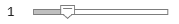1; % Offset in slots```

This figure displays an OFDM resource grid containing the configured SRS transmissions.

```hSRSGrid(carrier,srs,srs(1).SRSPeriod(1)*2, true); % Generate a multi-slot resource grid containing SRS title('SRS with Different Slot Offsets (SRSPeriod)') ylim([0 srs(1).NRBPerTransmission]);```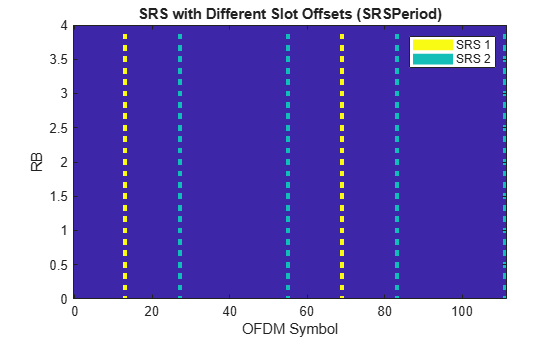Specify the number of SRS symbols and location in the slot to create orthogonal SRS transmissions.

First SRS configuration:

```srs = nrSRSConfig; srs(1).NumSRSSymbols =1; % Number of SRS symbols in a slot (1,2,4) srs(1).SymbolStart =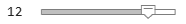12; % Time-domain position of the SRS in the slot. (8...13) for normal cyclic prefix (CP) and (6...11) for extended CP```

Second SRS configuration:

```srs(2) = srs(1); % Create a copy of the configured SRS srs(2).NumSRSSymbols =2; srs(2).SymbolStart =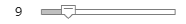9;```

This figure displays the OFDM resource grid containing the SRS transmissions.

```hSRSGrid(carrier,srs, 2, true); % Generate and display a 2-slot resource grid containing SRS title('SRS with Different Symbol Locations (SymbolStart)'); ylim([0 srs(1).NRBPerTransmission]);```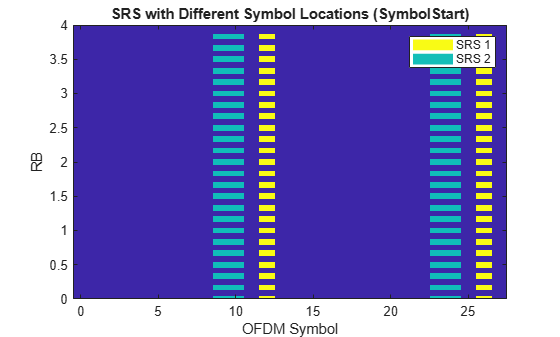#### Frequency-Domain Orthogonal SRS

You can create frequency-domain orthogonal SRS transmissions in multiple ways:

• Configure different comb offsets for different SRS by using the property `KBarTC`.

• Configure different frequency-hopping patterns using `CSRS`, `BSRS`, `BHop` and `NRRC`.

Configure a 10 MHz bandwidth carrier with 15 kHz SCS.

```carrier = nrCarrierConfig; carrier.NSizeGrid =52; carrier.SubcarrierSpacing =15;```

Specify the comb numbers and offsets to create orthogonal SRS transmissions.

First SRS configuration:

```srs = nrSRSConfig; srs(1).KTC =2; % Comb number (2,4). It indicates the allocation of the SRS every KTC subcarriers srs(1).KBarTC =1; % Comb offset (0...KTC-1)```

Second SRS configuration:

```srs(2) = srs(1); % Create a copy of the configured SRS srs(2).KTC =4; srs(2).KBarTC =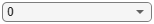0;```

This figure displays the OFDM resource grid containing the SRS transmissions.

```hSRSGrid(carrier,srs,2,true); title('SRS with Different Comb Offsets (KBarTC)') ylim([0 srs(1).NRBPerTransmission]);```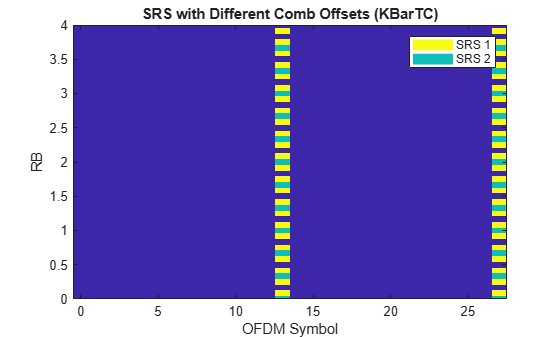Create frequency-domain orthogonal SRS configurations using different frequency-hopping patterns.

```srs = nrSRSConfig; srs.NumSRSSymbols =4; % Number of SRS symbols in a slot srs.SymbolStart =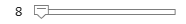8; % Allocate SRS in OFDM symbols 10:13```

Select the desired bandwidth configuration parameters, resource type and repetition factor.

```srs.CSRS =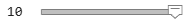10; % Bandwidth configuration C_SRS (0...63) srs.BSRS =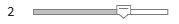2; % Bandwidth configuration B_SRS (0...3) srs.BHop =0; % Frequency hopping configuration (0...3). Set BHop >= BSRS to disable frequency hopping srs.ResourceType ="periodic"; % ('periodic','semi-persistent','aperiodic') srs.Repetition =1; % Number of equal consecutive SRS transmissions (1,2,4). It must be lower than NumSRSSymbols```

This example calculates the number of orthogonal SRS sequences that can be configured by `NRRC` and creates frequency non-overlapping SRS configurations. The figure displays the OFDM resource grid containing the SRS transmissions.

```NRRC = num2cell(hNRRCSet(srs)); srs(2:length(NRRC)) = srs(1); % Create N-1 copies of the configured SRS [srs(:).NRRC] = deal(NRRC{:}); % Assign the appropriate NRRC to each SRS configuration hSRSGrid(carrier,srs, 3, true); % Generate and display a 3-slot resource grid containing SRS title('SRS with Different Frequency-Hopping Patterns'); ylim([0 srs(1).NRBPerTransmission*hSRSNumberOfSubbandsOrHoppingPatterns(srs(1))]);```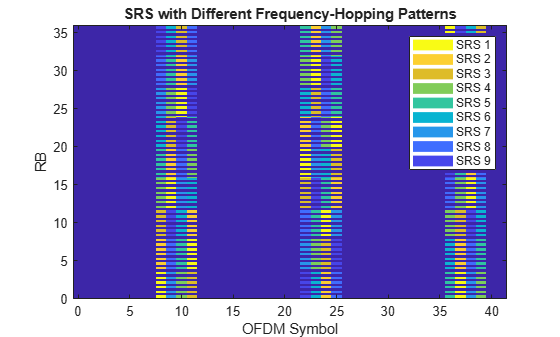```N = hSRSNumberOfSubbandsOrHoppingPatterns(srs(1)); if srs(1).BHop < srs(1).BSRS % Frequency-hopping cases fprintf('Number of unique frequency-hopping patterns (configurable by NRRC < %d): %d.', N*srs(1).NRBPerTransmission/4, N); else fprintf('Number of unique subbands (configurable by NRRC < %d): %d.', N*srs(1).NRBPerTransmission/4,N); end```
```Number of unique frequency-hopping patterns (configurable by NRRC < 9): 9. ```

Note that the SRS transmissions are never overlapping regardless of the `Repetition` factor and `ResourceType` (`aperiodic` disables inter-slot frequency hopping). Disable frequency hopping by setting `BHop >= BSRS` and observe that the different SRS are still allocated to different subbands.

#### Cyclic-Shift Orthogonal SRS

This section generates multiple SRS allocated to the same time and frequency resources (OFDM symbols and subcarriers) but different time-domain cyclic shifts. Due to the properties of Zadoff-Chu sequences, this configuration produces orthogonal SRS. To demonstrate the orthogonality among the configured SRS, this section performs CP-OFDM modulation and calculates the cross-correlation of the time-domain waveforms.

```% Configure a 10 MHz bandwidth carrier with 15 kHz SCS. carrier = nrCarrierConfig; carrier.NSizeGrid = 52; carrier.SubcarrierSpacing = 15; % Create a full-band SRS srs = nrSRSConfig; srs.CSRS = hSRSBandwidthConfiguration(srs,carrier.NSizeGrid); % All SRS share the same physical resources, but they are configured with % different cyclic shifts. for i = 1:8 srs(i) = srs(1); srs(i).CyclicShift = i-1; end % Create a resource grid containing SRS numSlots = 1; % Number of slots to generate for ich = length(srs):-1:1 slotGrid{ich} = hSRSGrid(carrier,srs(ich),numSlots); end % Get OFDM modulation related information ofdmInfo = nrOFDMInfo(carrier); % OFDM modulation nsrs = length(srs); % Number of SRS waveforms numSamples = numSlots*ofdmInfo.SampleRate/1000/carrier.SlotsPerSubframe; txWaveform = zeros(numSamples,srs(1).NumSRSPorts,nsrs); for i = 1:nsrs txWaveform(:,:,i) = nrOFDMModulate(carrier,slotGrid{i}); end % Cross correlation of SRS waveforms generated with different cyclic shifts txWaveform = reshape(txWaveform,[],nsrs*srs(1).NumSRSPorts); C = txWaveform'*txWaveform; srsCorr = C./diag(C);```

This figure shows the time-domain cross-correlation of the SRS waveforms with different cyclic shifts.

```imagesc(abs(srsCorr)) title('Correlation of SRS with Different CyclicShift') xlabel('SRS configuration'); ylabel('SRS configuration'); colorbar```The low correlations among SRS waveforms generated using different time-domain cyclic shifts show their orthogonality.

`disp('Absolute value of correlation matrix: '); disp(' '); disp(abs(srsCorr));`
```Absolute value of correlation matrix: Columns 1 through 7 1.0000 0.0027 0.0021 0.0008 0.0003 0.0010 0.0007 0.0027 1.0000 0.0030 0.0006 0.0006 0.0001 0.0006 0.0021 0.0030 1.0000 0.0041 0.0007 0.0008 0.0003 0.0008 0.0006 0.0041 1.0000 0.0040 0.0004 0.0009 0.0003 0.0006 0.0007 0.0040 1.0000 0.0039 0.0002 0.0010 0.0001 0.0008 0.0004 0.0039 1.0000 0.0039 0.0007 0.0006 0.0003 0.0009 0.0002 0.0039 1.0000 0.0038 0.0005 0.0008 0.0000 0.0009 0.0003 0.0039 Column 8 0.0038 0.0005 0.0008 0.0000 0.0009 0.0003 0.0039 1.0000 ```

### Summary and Further Exploration

This example describes how to generate and map SRS sequences into an OFDM carrier resource grid, and how to generate the corresponding waveform for multiple carrier and SRS configurations. The example highlights the relationship between SRS configuration parameters and their effects on both the resource grid and SRS waveform properties. For example:

• The bandwidth allocated to the SRS generally increases with `CSRS` and decreases with `BSRS`. For frequency-hopping configurations, the instantaneous bandwidth and hopping bandwidth decrease with `BSRS` and `BHop`, respectively.

• The frequency position of the SRS depends on the `FrequencyStart` and `NRRC` parameters. `NRRC` allows to select different SRS subbands and frequency-hopping patterns.

• `BHop < BSRS` generally produces frequency hopping, but it is not guaranteed. However, `BHop >= BSRS` is sufficient to disable frequency hopping.

• Inter-slot frequency hopping is active only for `periodic` and `semi-persistent` resource types.

• Time-, frequency-, and sequence-related parameters can be used to create orthogonal SRS transmissions.

The example also demonstrates how to extract useful information from both the SRS bandwidth configuration table and read-only properties, such as, the number of unique SRS subbands and frequency-hopping patterns configurable by `NRRC`.

The SRS sequence generation for multiport configurations employs the orthogonalization mechanism presented in Cyclic-Shift Orthogonal SRS. To verify the orthogonality across multiple ports, configure a multi-port SRS, generate the symbols and indices, map the symbols into a resource grid, generate the SRS waveforms, and compute the cross-correlation between waveforms generated for different ports.

### References

 3GPP TS 38.211. “NR; Physical channels and modulation.” 3rd Generation Partnership Project; Technical Specification Group Radio Access Network.

 3GPP TS 38.331. “NR; Radio Resource Control (RRC) protocol specification.” 3rd Generation Partnership Project; Technical Specification Group Radio Access Network.

### Local functions

This example uses these local functions:

```function [csrs,bsrs] = hSRSBandwidthConfiguration(srs, NRB) % [CSRS,BSRS] = hBandwidthConfiguration(SRS, NRB) returns the SRS % bandwidth configuration parameters CSRS and BSRS required to transmit an % SRS in a bandwidth specified by NRB. The function calculates CSRS and % BSRS considering the SRS properties FrequencyStart and NRRC, so the % available bandwidth NRB is reduced by the frequency origin of the SRS. % For frequency hopping cases, the value of BSRS returned is equal to that % of the BSRS property in the input SRS configuration object. f0 = hSRSFrequencyOrigin(srs); NRB = NRB - f0; if NRB < 4 error('The available bandwidth is not sufficient to allocate an SRS transmission. Increase the carrier bandwidth or configure the SRS in a lower frequency.' ) end % For frequency hopping configurations if srs.BHop >= srs.BSRS && nargout == 2 % No frequency hopping and BSRS is requested BSRS = 0:3; else BSRS = 0; end % Initialize gap between SRS frequency allocation and carrier bandwidth NRBGap = NRB; % Find the appropriate CSRS for each BSRS that minimizes the gap only % in non-hopping cases. For freq. hopping, find the value of CSRS only. for b = BSRS % NRB allocated to the SRS for BSRS = b and all CSRS srsNRBb = srs.BandwidthConfigurationTable{:,2*(b+1)}; mSRSbMax = max( srsNRBb( srsNRBb <= NRB )); % Calculate gap between SRS allocation and carrier bandwidth gap = NRB - mSRSbMax; if gap < NRBGap csrs = srs.BandwidthConfigurationTable{ srsNRBb == mSRSbMax ,1}; bsrs = b; NRBGap = gap; end end csrs = csrs(1); if srs.BHop < bsrs bsrs = srs.BSRS; end end function out = hSRSNumberOfSubbandsOrHoppingPatterns(srs) % N = hSRSNumberOfSubbandsOrHoppingPatterns(SRS) returns the number of % unique SRS subbands or frequency-hopping patterns N configurable by the % SRS property NRRC. An SRS subband is the frequency band allocated for SRS % in a given OFDM symbol (See SRS property NRBPerTransmission). N is % calculated using TS 38.211 Table 6.4.1.4.3-1 for the bandwidth % configuration parameters CSRS, BSRS, and BHop specified in the SRS % configuration object SRS. bwct = nrSRSConfig.BandwidthConfigurationTable{:,:}; if srs.BHop < srs.BSRS % Number of unique frequency-hopping patterns b0 = srs.BHop+1; else % Number of unique SRS subbands b0 = 0; end out = prod(bwct(srs.CSRS+1,2*(b0:srs.BSRS)+3)); end function [Grid,dispGrid] = hSRSGrid(carrier,srs,Duration,displayGrid,chplevels) % [GRID,DISPGRID] = hSRSGrid(CARRIER,SRS,DURATION,DISPLAYGRID,CHPLEVELS) % returns a multi-slot OFDM resource grid GRID containing a set of sounding % reference signals in a carrier, as specified by the configuration objects % CARRIER and SRS. This function also returns a scaled version of the grid % used for display purposes. The optional input DURATION (Default 1) % specifies the number of slots of the generated grid. The resource grid % can be displayed using the optional input DISPLAYGRID (Default false). % CHPLEVELS specifies the channel power levels for display purposes only % and it must be of the same size as SRS. numSRS = length(srs); if nargin < 5 chplevels = 1:-1/numSRS:1/numSRS; if nargin < 4 displayGrid = false; if nargin < 3 Duration = 1; end end end SymbolsPerSlot = carrier.SymbolsPerSlot; emptySlotGrid = nrResourceGrid(carrier,max([srs(:).NumSRSPorts])); % Initialize slot grid % Create the SRS symbols and indices and populate the grid with the SRS symbols Grid = repmat(emptySlotGrid,1,Duration); dispGrid = repmat(emptySlotGrid,1,Duration); % Frame-size grid for display for ns = 0:Duration-1 slotGrid = emptySlotGrid; dispSlotGrid = emptySlotGrid; % Slot-size grid for display for ich = 1:numSRS srsIndices = nrSRSIndices(carrier,srs(ich)); srsSymbols = nrSRS(carrier,srs(ich)); slotGrid(srsIndices) = srsSymbols; dispSlotGrid(srsIndices) = chplevels(ich)*srsSymbols; % Scale the SRS for display only end OFDMSymIdx = ns*SymbolsPerSlot + (1:SymbolsPerSlot); Grid(:,OFDMSymIdx,:) = slotGrid; dispGrid(:,OFDMSymIdx,:) = dispSlotGrid; carrier.NSlot = carrier.NSlot+1; end if displayGrid plotGrid(dispGrid(:,:,1),chplevels,"SRS " + (1:numSRS)'); end end function varargout = plotGrid(Grid,chplevels,leg) % plotGrid(GRID, CHPLEVEL,LEG) displays a resource grid GRID containing % channels or signals at different power levels CHPLEVEL and create a % legend for these using a cell array of character vector LEG if nargin < 3 leg = {'SRS'}; if nargin < 2 chplevels = 1; end end cmap = colormap(gcf); chpscale = length(cmap); % Scaling factor h = figure; image(0:size(Grid,2)-1,(0:size(Grid,1)-1)/12,chpscale*abs(Grid(:,:,1))); % Multiplied with scaling factor for better visualization axis xy; title('Carrier Grid Containing SRS') xlabel('OFDM Symbol'); ylabel('RB'); clevels = chpscale*chplevels(:); N = length(clevels); L = line(ones(N),ones(N),'LineWidth',8); % Generate lines % Index the color map and associate the selected colors with the lines set(L,{'color'},mat2cell(cmap( min(1+fix(clevels),length(cmap) ),:),ones(1,N),3)); % Set the colors according to cmap % Create legend legend(leg(:)); if nargout > 0 varargout = {h}; end end function hSRSAnnotations(carrier,srs) % hSRSAnnotations(carrier,srs) adds annotation to the current figure % indicating the frequency origin of the SRS and the number of RB used per % OFDM symbol for the configuration objects CARRIER and SRS. % Calculate the frequency origin of the first SRS symbol f0 = hSRSFrequencyOrigin(srs); hold on; hfig = gcf; set(hfig,'Units','Normalized'); Sym0 = srs.SymbolStart-0.5; if isnumeric(srs.SRSPeriod) Sym0 = srs.SRSPeriod(2)*carrier.SymbolsPerSlot + srs.SymbolStart-0.5; end IP = get(gca,'Position'); % Y-coordinate in the current axes of the SRS freq position f0 Yf0 = f0/carrier.NSizeGrid*IP(4)+IP(2); Xc = Sym0/((carrier.NSlot+1)*carrier.SymbolsPerSlot)*IP(3)+IP(1); % Add annotation to the figure including f0 in RB if f0/carrier.NSizeGrid > 0.08 % Only plot f0 when there is enough space in the y-axis % Create doublearrow for F0 YMin = IP(2); annotation(hfig,'doublearrow',Xc*[1 1], [YMin Yf0],... 'Color',[1 0.4 0.15]); % Text for F0 str = sprintf('\$\$F_0 = %d \$\$ RB', f0); Ystr = f0/carrier.NSizeGrid/2; text(gca,(Xc-IP(1))/IP(3)-0.25, Ystr,str,... 'Color',[1 0.4 0.15],'FontSize',14, ... 'Units','Normalized','Interpreter','latex'); end % Create doublearrow from f0 and spanning the SRS bandwidth Yf1 = Yf0 + srs.NRBPerTransmission/carrier.NSizeGrid*IP(4); annotation(hfig,'doublearrow',Xc*[1 1], [Yf0 Yf1],... 'Color',[1 0.4 0.15]); % Text for NRBt str = sprintf('\$\$\\textrm{NRB}_T = %d \$\$ RB', srs.NRBPerTransmission); Ystr = (f0 + 0.5*srs.NRBPerTransmission)/carrier.NSizeGrid; text(gca,(Xc-IP(1))/IP(3)-0.32, Ystr ,str,... 'Color',[1 0.4 0.15],'FontSize',14, ... 'Units','Normalized','Interpreter','latex'); end function f0 = hSRSFrequencyOrigin(srs) % Calculate the frequency origin of the first SRS symbol in a slot NSBTable = hSRSNumberOfSubbandsOrHoppingPatterns(srs); NRBt = srs.NRBPerTransmission; % Origin of the SRS in frequency in RB f0 = srs.FrequencyStart + NRBt*mod(floor(4*srs.NRRC/NRBt),NSBTable); end function [NRRC,NRB] = hNRRCSet(srs) % Calculate the values of NRRC that generate a unique set of orthogonal SRS in frequency if srs.BHop < srs.BSRS % Frequency-hopping cases NRB = srs(1).NRB; % Hopping bandwidth else NRB = nrSRSConfig.BandwidthConfigurationTable{srs(1).CSRS+1,2}; end % Number of frequency-hopping patterns or SRS subbands depending on the values of BSRS and BHop N = hSRSNumberOfSubbandsOrHoppingPatterns(srs); NRRC = NRB/4*(0:N-1)/N; end```

 3GPP TS 38.211. “NR; Physical channels and modulation.” 3rd Generation Partnership Project; Technical Specification Group Radio Access Network.